﻿ 基于微穿孔板的通风管道噪声自适应控制算法研究 Study on Adaptive Control Algorithm of Ventilation Duct Noise Based on Micro-Perforated Pane

Open Journal of Acoustics and Vibration
Vol. 06  No. 03 ( 2018 ), Article ID: 26692 , 11 pages
10.12677/OJAV.2018.63011

Study on Adaptive Control Algorithm of Ventilation Duct Noise Based on Micro-Perforated Pane

Weiguang Zheng, Zeyu Lin, Rongjiang Tang, Peng Xu

School of Mechanical and Electrical Engineering, Guilin University of Electronic Technology, Guilin Guangxi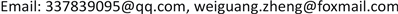Received: Aug. 17th, 2018; accepted: Aug. 30th, 2018; published: Sep. 5th, 2018ABSTRACT

Pipeline noise includes low, medium and high frequency noise. In order to realize the active control of broadband pipeline noise, this paper combined with impedance compound muffler structure and active noise reduction method, proposed resonance sound absorption structure weaken noise source of the sound radiation and combined with the impedance characteristics of microperforated panel acoustic absorption mode optimization matching method. This paper combines two adaptive control algorithms to study the sound absorption law of pipelines, proposes different active noise reduction schemes, simulates the iterative waveforms of different algorithms, and summarizes the influence of the parameters of its adaptive control algorithm on the sound absorption effect. The feed forward control system of the pipe sound absorption platform was established to further verify the conclusion of the experiment.

Keywords:Microperforated Plate Sound Absorption Structure, Adaptive Filter, FXLMS Algorithm, RLS Algorithm1. 引言

2. 前馈管道噪声控制模型

2.1. 阻抗复合式消声结构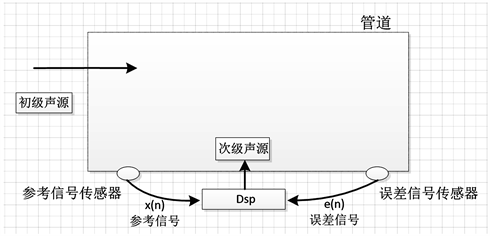Figure 1. The system model of feed-forward ANC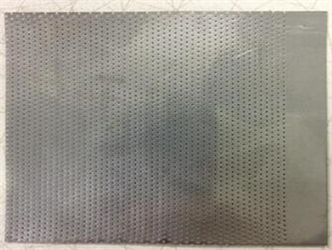(a)(b)

Figure 2. The structure diagram of micro-perforated plate sound absorption

2.2. 主动控制策略

$\omega \left(n+1\right)=\omega \left(n\right)-2\upsilon x\left(n\right)e\left(n\right)$ (1)

1) 确定L、 $\upsilon$$\omega \left(0\right)$ ，其中L为滤波器的长度， $\upsilon$ 为步长， $\omega \left(0\right)$ 为时间n = 0时的滤波器的初始化值；

2) 计算自适应滤波器的输出y(n)；

$y\left(n\right)=\sum _{l=0}^{L-1}{\omega }_{l}\left(n\right)x\left(n-l\right)$ (2)

3) 计算误差信号e(n);

$e\left(n\right)=x\left(n\right)-y\left(n\right)$ (3)

4) 通过FXLMS算法更新自适应滤波器的权重；

${\omega }_{l}\left(n+1\right)={\omega }_{l}\left(n\right)+\upsilon x\left(n-l\right)e\left(n\right),\text{\hspace{0.17em}}\text{\hspace{0.17em}}l=0,1,\cdots ,L-1$ (4)

h = adaptfilt.algorithm (input 1, input 2, ∙∙∙∙∙∙) (5)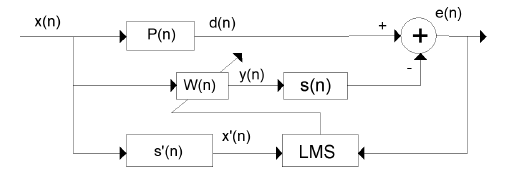Figure 3. The block diagram of adaptive filtering algorithm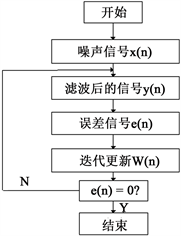Figure 4. The flow diagram of FXLMS algorithm program

h = dsp.FilteredXLMSFilter (l, 'StepSize', mu, 'LeakageFactor', ∙∙∙∙∙∙ 1, 'SecondaryPathCoefficients', b) (6)

3. 前馈系统最优控制策略

3.1. 主动控制策略仿真

3.2. 前馈控制系统设计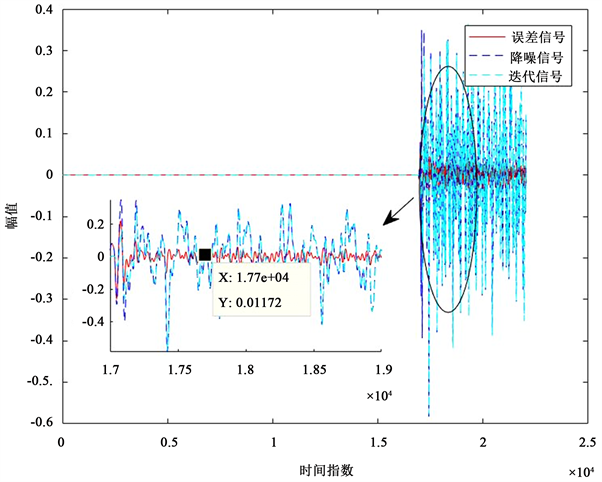Figure 5. Adaptive filtering of FXLMS algorithm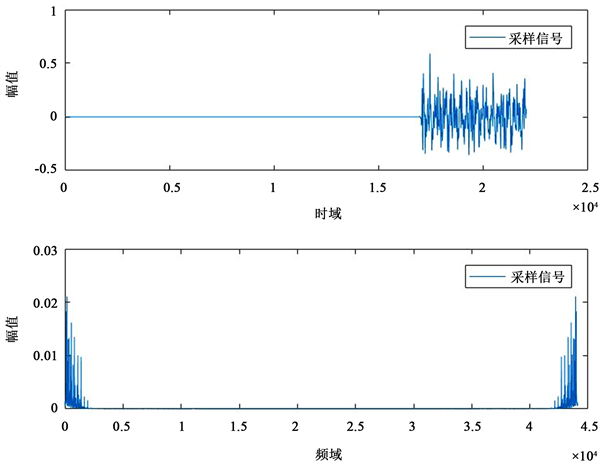Figure 6. The original noise signal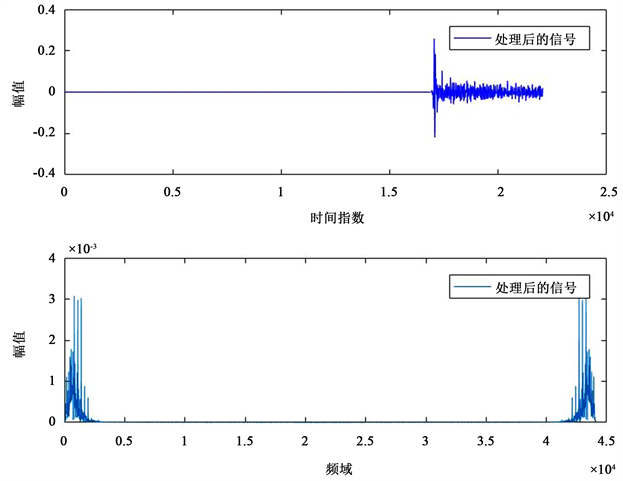(a)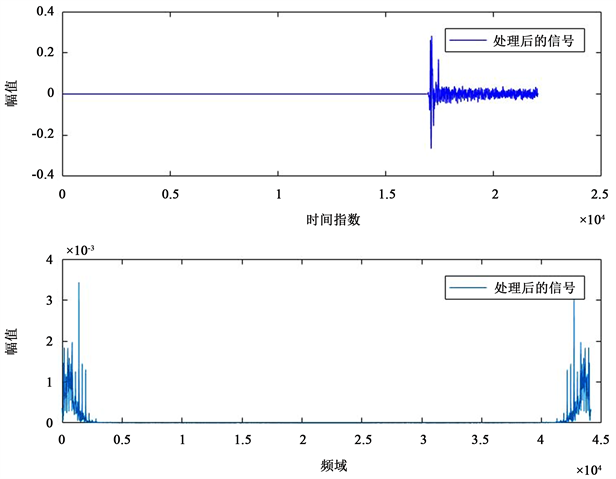(b)

Figure 7. The noise reduction effect of two adaptive algorithms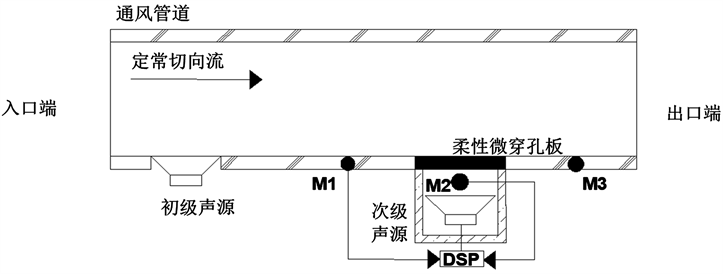Figure 8. The system diagram of feed-forward adaptive control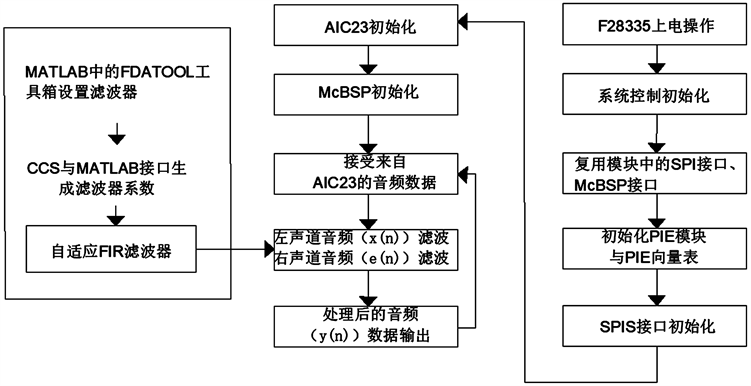Figure 9. The software block diagram of control system

3.3. 前馈控制系统实验

4. 总结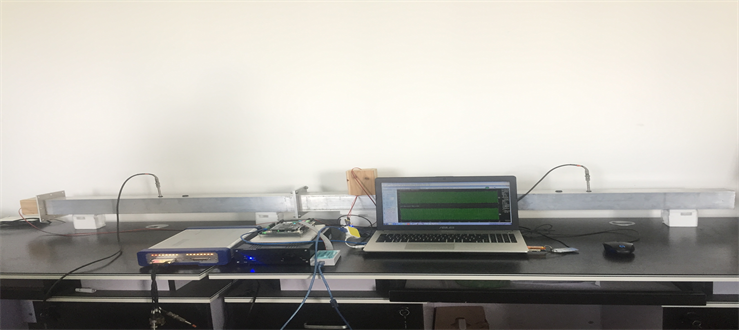Figure 10. Physical drawings of the experimental system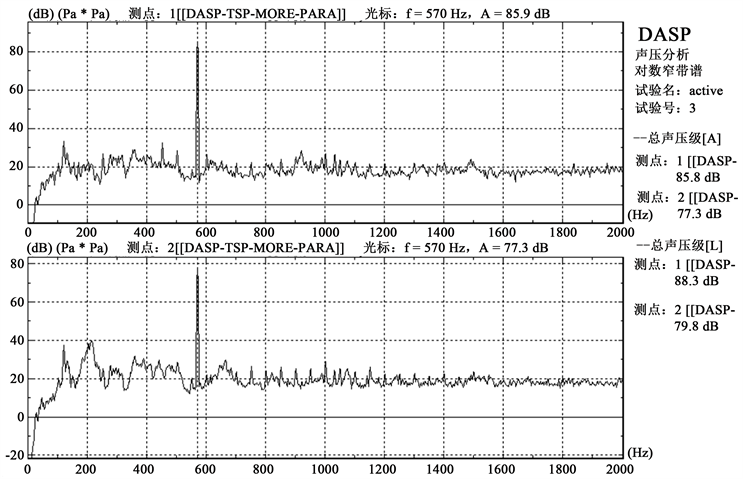(a)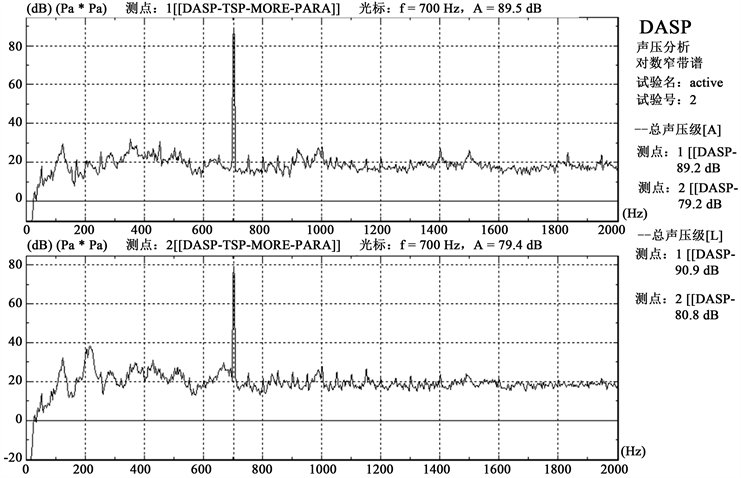(b)

Figure 11. The sound pressure level at the measuring point under the primary noise

1) 在基于柔性微穿孔板的被动噪声控制下，加入主动噪声控制的方法，有利于低、中噪声频率的消除，使得整个管道实现宽频带噪声的控制；

2) 在主动控制策略方面，自适应算法的效果与自适应权重(自适应滤波系数)的选取有关，为了得到较好的减噪效果，通常采用编程的方法获取；

3) 通过比较自适应滤波的减噪效果，可以看出基于RLS算法的主被动控制系统具有更好的宽频带消声，但同时计算量也会增大。

Study on Adaptive Control Algorithm of Ventilation Duct Noise Based on Micro-Perforated Pane[J]. 声学与振动, 2018, 06(03): 88-98. https://doi.org/10.12677/OJAV.2018.63011

1. 1. 陈克安. 有源噪声控制[M]. 北京: 国防工业出版社, 2003.

2. 2. Sellen, N., Cuesta, M. and Galland, M.A. (2006) Noise Reduction in a Flow Duct: Implementation of a Hybrid Passive/Active Solution. Journal of Sound & Vibration, 297, 492-511. https://doi.org/10.1016/j.jsv.2006.03.049

3. 3. 赵栋, 李凯翔, 张飞, 庞彦宾. 主动噪声控制系统在C6000DSP上的设计与实现[J]. 测控技术, 2017, 36(1): 63-66.

4. 4. Sen M. Kuo, Bob H. Lee, Wenshun Tian. 实时数字信号处理: 实践方法与应用[M]. 北京: 清华大学出版社, 2012.

5. 5. 孙琳. 基于DSP的窄带主动噪声控制系统实现[J]. 仪器仪表学报, 2011, 32(2): 252-257.

6. 6. 姜占才, 孙燕, 王得芳. 基于谱减和LMS的自适应语音增强[J]. 计算机工程与应用, 2012, 48(7): 42-45.